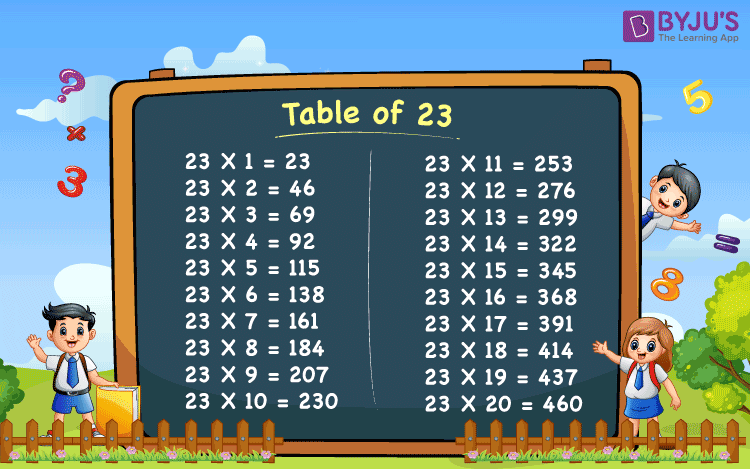# Table of 23

Table of 23 gives the repeated addition of the number 23 for the “n” number of times. We are learning maths basic principles and fundamentals, since our childhood in primary and junior classes. Maths tables are also one of the fundamentals of Mathematics. Along with the table of 23, tables of 24, 25, 26, 27, up to 30, should be memorized by students to do calculations quickly.

For example, there are 4 rooms in a house each has 23 cupboards in it. How to calculate the total number of cupboards in all four rooms? We need to add 23 for 4 times repeatedly to get the answer, i.e. 23+23+23+23 = 92. Therefore, there is a total of 92 cupboards. Or we can also calculate it like 4 times of 23, 4 x 23 = 92.

## Table of 23 ChartTable of 23 is useful not only for students of schools or colleges but also for the candidates appearing for competitive exams conducted at the state level, national level, or international level. These exams have maths aptitude questions which consist of multiplication calculations. Aspiring candidates should learn the Maths tables to solve the calculations easily and quickly.

## Multiplication Table of 23

Let us have a look at the table of number 23 for 1 to 20 times.

 23 x 1 = 23 23 x 2 = 46 23 x 3 = 69 23 x 4 = 92 23 x 5 = 115 23 x 6 = 138 23 x 7 = 161 23 x 8 = 184 23 x 9 = 207 23 x 10 = 230 23 x 11 = 253 23 x 12 = 276 23 x 13 = 299 23 x 14 = 322 23 x 15 = 345 23 x 16 = 368 23 x 17 = 391 23 x 18 = 414 23 x 19 = 437 23 x 20 = 460

In the table of 23, you can see, 23 is the multiplicand and the numbers from 1 to 20 are multipliers. So, in the table of 23, the number 23 is multiplied by the other numbers to get the results.

### 23 Times Table Examples

Example1:

Evaluate 3 plus 2 times 23.

Solution:

3 plus 2 times 23 = 3+2(23)

= 3+46

=49

Hence, 3 plus 2 times 23 is 49.

Example 2:

What would be the answer if 23 is added to 5 times 23.

Solution:

23 added to 5 times 23 means 23+5(23)

= 23 + 115

= 138

Hence, 23 added to 5 times 23 is 138.

 More Maths Table Table Of 20 Table Of 21 Table Of 22 Table Of 24 Table Of 25 Table Of 26 Table Of 27 Table Of 28 Table Of 29 Table Of 30

Stay tuned with BYJU’S – The Learning App and download the app to learn all Maths-related concepts quickly by watching more exciting videos.

## Frequently Asked Questions on Tables of 23

Q1

### What is 23 times table?

The multiplication table of 23 or 23 times table is the multiplication of the number 23 with other natural numbers to get the result.

Q2

### Write the table of 23 from 1 to 10.

The table of 23 from 1 to 10 are:
23 × 1 = 23
23 × 2 = 46
23 × 3 = 69
23 × 4 = 92
23 × 5 = 115
23 × 6 = 138
23 × 7 = 161
23 × 8 = 184
23 × 9 = 207
23 × 10 = 230

Q3

### Write the 23 times table from 11 to 20.

The table of 23 from 11 to 20 are:
23 × 11 = 253
23 × 12 = 276
23 × 13 = 299
23 × 14 = 322
23 × 15 = 345
23 × 16 = 368
23 × 17 = 391
23 × 18 = 414
23 × 19 = 437
23 × 20 = 460

Test your knowledge on Table Of 23